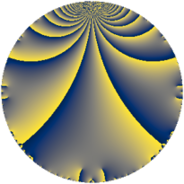# Properties

 Label 2475.2.a.aLevel $2475$ Weight $2$ Character orbit 2475.a Self dual yes Analytic conductor $19.763$ Analytic rank $0$ Dimension $1$ CM no Inner twists $1$

# Related objects

## Newspace parameters

 Level: $$N$$ $$=$$ $$2475 = 3^{2} \cdot 5^{2} \cdot 11$$ Weight: $$k$$ $$=$$ $$2$$ Character orbit: $$[\chi]$$ $$=$$ 2475.a (trivial)

## Newform invariants

 Self dual: yes Analytic conductor: $$19.7629745003$$ Analytic rank: $$0$$ Dimension: $$1$$ Coefficient field: $$\mathbb{Q}$$ Coefficient ring: $$\mathbb{Z}$$ Coefficient ring index: $$1$$ Twist minimal: no (minimal twist has level 11) Fricke sign: $$-1$$ Sato-Tate group: $\mathrm{SU}(2)$

## $q$-expansion

 $$f(q)$$ $$=$$ $$q - 2q^{2} + 2q^{4} + 2q^{7} + O(q^{10})$$ $$q - 2q^{2} + 2q^{4} + 2q^{7} - q^{11} - 4q^{13} - 4q^{14} - 4q^{16} - 2q^{17} + 2q^{22} - q^{23} + 8q^{26} + 4q^{28} + 7q^{31} + 8q^{32} + 4q^{34} - 3q^{37} + 8q^{41} + 6q^{43} - 2q^{44} + 2q^{46} + 8q^{47} - 3q^{49} - 8q^{52} - 6q^{53} - 5q^{59} + 12q^{61} - 14q^{62} - 8q^{64} + 7q^{67} - 4q^{68} + 3q^{71} - 4q^{73} + 6q^{74} - 2q^{77} - 10q^{79} - 16q^{82} - 6q^{83} - 12q^{86} - 15q^{89} - 8q^{91} - 2q^{92} - 16q^{94} + 7q^{97} + 6q^{98} + O(q^{100})$$

## Embeddings

For each embedding $$\iota_m$$ of the coefficient field, the values $$\iota_m(a_n)$$ are shown below.

For more information on an embedded modular form you can click on its label.

Label $$\iota_m(\nu)$$ $$a_{2}$$ $$a_{3}$$ $$a_{4}$$ $$a_{5}$$ $$a_{6}$$ $$a_{7}$$ $$a_{8}$$ $$a_{9}$$ $$a_{10}$$
1.1
 0
−2.00000 0 2.00000 0 0 2.00000 0 0 0
 $$n$$: e.g. 2-40 or 990-1000 Significant digits: Format: Complex embeddings Normalized embeddings Satake parameters Satake angles

## Atkin-Lehner signs

$$p$$ Sign
$$3$$ $$-1$$
$$5$$ $$1$$
$$11$$ $$1$$

## Inner twists

This newform does not admit any (nontrivial) inner twists.

## Twists

By twisting character orbit
Char Parity Ord Mult Type Twist Min Dim
1.a even 1 1 trivial 2475.2.a.a 1
3.b odd 2 1 275.2.a.b 1
5.b even 2 1 99.2.a.d 1
5.c odd 4 2 2475.2.c.a 2
12.b even 2 1 4400.2.a.i 1
15.d odd 2 1 11.2.a.a 1
15.e even 4 2 275.2.b.a 2
20.d odd 2 1 1584.2.a.g 1
33.d even 2 1 3025.2.a.a 1
35.c odd 2 1 4851.2.a.t 1
40.e odd 2 1 6336.2.a.bu 1
40.f even 2 1 6336.2.a.br 1
45.h odd 6 2 891.2.e.k 2
45.j even 6 2 891.2.e.b 2
55.d odd 2 1 1089.2.a.b 1
60.h even 2 1 176.2.a.b 1
60.l odd 4 2 4400.2.b.h 2
105.g even 2 1 539.2.a.a 1
105.o odd 6 2 539.2.e.h 2
105.p even 6 2 539.2.e.g 2
120.i odd 2 1 704.2.a.h 1
120.m even 2 1 704.2.a.c 1
165.d even 2 1 121.2.a.d 1
165.o odd 10 4 121.2.c.e 4
165.r even 10 4 121.2.c.a 4
195.e odd 2 1 1859.2.a.b 1
240.t even 4 2 2816.2.c.f 2
240.bm odd 4 2 2816.2.c.j 2
255.h odd 2 1 3179.2.a.a 1
285.b even 2 1 3971.2.a.b 1
345.h even 2 1 5819.2.a.a 1
420.o odd 2 1 8624.2.a.j 1
435.b odd 2 1 9251.2.a.d 1
660.g odd 2 1 1936.2.a.i 1
1155.e odd 2 1 5929.2.a.h 1
1320.b odd 2 1 7744.2.a.k 1
1320.u even 2 1 7744.2.a.x 1

By twisted newform orbit
Twist Min Dim Char Parity Ord Mult Type
11.2.a.a 1 15.d odd 2 1
99.2.a.d 1 5.b even 2 1
121.2.a.d 1 165.d even 2 1
121.2.c.a 4 165.r even 10 4
121.2.c.e 4 165.o odd 10 4
176.2.a.b 1 60.h even 2 1
275.2.a.b 1 3.b odd 2 1
275.2.b.a 2 15.e even 4 2
539.2.a.a 1 105.g even 2 1
539.2.e.g 2 105.p even 6 2
539.2.e.h 2 105.o odd 6 2
704.2.a.c 1 120.m even 2 1
704.2.a.h 1 120.i odd 2 1
891.2.e.b 2 45.j even 6 2
891.2.e.k 2 45.h odd 6 2
1089.2.a.b 1 55.d odd 2 1
1584.2.a.g 1 20.d odd 2 1
1859.2.a.b 1 195.e odd 2 1
1936.2.a.i 1 660.g odd 2 1
2475.2.a.a 1 1.a even 1 1 trivial
2475.2.c.a 2 5.c odd 4 2
2816.2.c.f 2 240.t even 4 2
2816.2.c.j 2 240.bm odd 4 2
3025.2.a.a 1 33.d even 2 1
3179.2.a.a 1 255.h odd 2 1
3971.2.a.b 1 285.b even 2 1
4400.2.a.i 1 12.b even 2 1
4400.2.b.h 2 60.l odd 4 2
4851.2.a.t 1 35.c odd 2 1
5819.2.a.a 1 345.h even 2 1
5929.2.a.h 1 1155.e odd 2 1
6336.2.a.br 1 40.f even 2 1
6336.2.a.bu 1 40.e odd 2 1
7744.2.a.k 1 1320.b odd 2 1
7744.2.a.x 1 1320.u even 2 1
8624.2.a.j 1 420.o odd 2 1
9251.2.a.d 1 435.b odd 2 1

## Hecke kernels

This newform subspace can be constructed as the intersection of the kernels of the following linear operators acting on $$S_{2}^{\mathrm{new}}(\Gamma_0(2475))$$:

 $$T_{2} + 2$$ $$T_{7} - 2$$ $$T_{29}$$

## Hecke characteristic polynomials

$p$ $F_p(T)$
$2$ $$2 + T$$
$3$ $$T$$
$5$ $$T$$
$7$ $$-2 + T$$
$11$ $$1 + T$$
$13$ $$4 + T$$
$17$ $$2 + T$$
$19$ $$T$$
$23$ $$1 + T$$
$29$ $$T$$
$31$ $$-7 + T$$
$37$ $$3 + T$$
$41$ $$-8 + T$$
$43$ $$-6 + T$$
$47$ $$-8 + T$$
$53$ $$6 + T$$
$59$ $$5 + T$$
$61$ $$-12 + T$$
$67$ $$-7 + T$$
$71$ $$-3 + T$$
$73$ $$4 + T$$
$79$ $$10 + T$$
$83$ $$6 + T$$
$89$ $$15 + T$$
$97$ $$-7 + T$$